Top k算法模式，你值得拥有！！！

# 二、算法模式

Top k 算法模式就利用Heap（堆）来跟踪给定集合的前K个元素，从而一次性的处理一个给定元素集中前K个元素的问题。

## 2.1、工作模式

1、根据给定元素集合，将k个元素插入到min_heap或者max_heap中

2、利用heap的性质进行迭代的处理剩余的元素。例如你需要找到一个给定元素集合中的前k个大的元素，则可以先将前k元素建立min_heap，然后遍历后续的元素，当pop_heap元素小于当前遍历的元素T[index]，则将堆顶元素换成当前遍历的元素T[index]，再维持min_heap。最后便可以得到一个前k大的元素。如下图：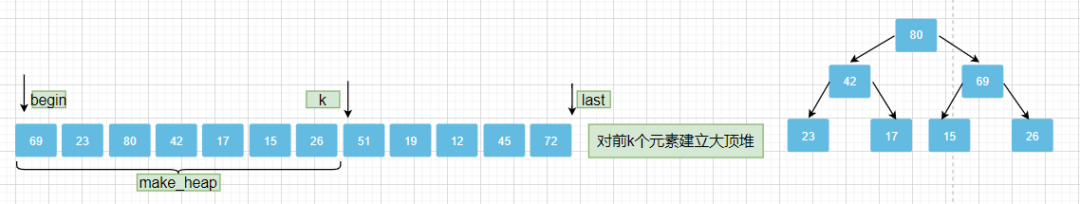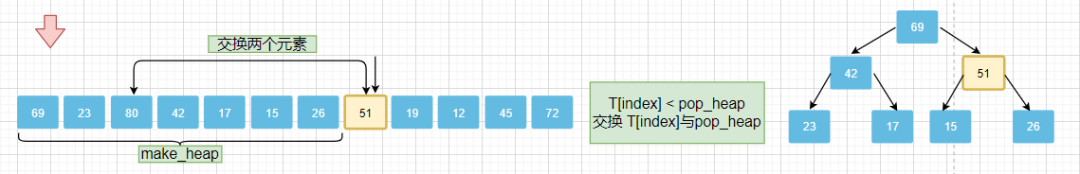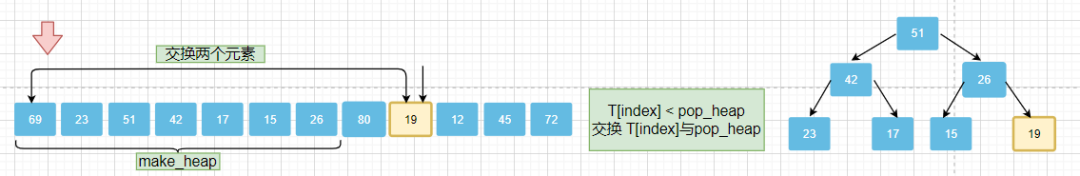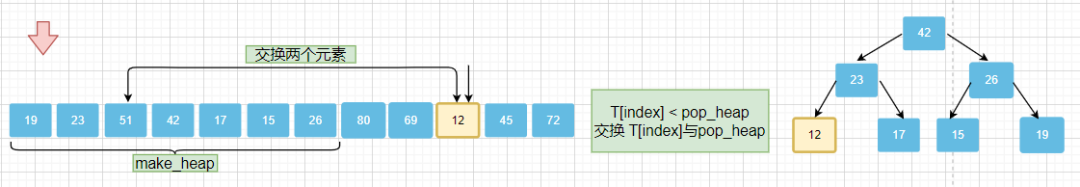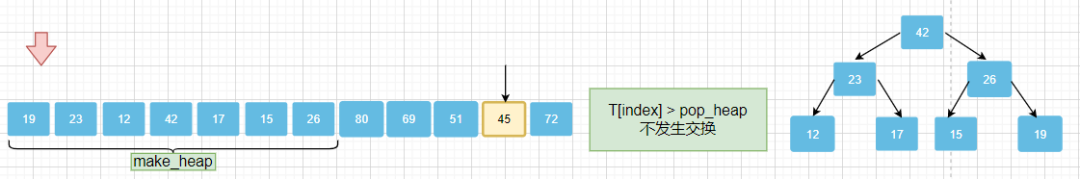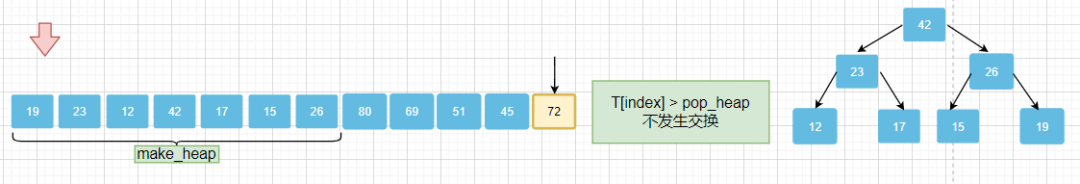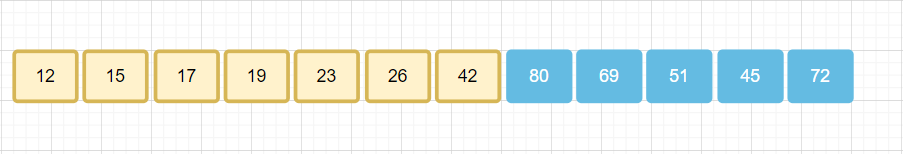1、寻找给定集合中最大的/最小的/最常出现的k个元素
2、对给定集合进行排序从而找到一个确定元素，如寻找第k个元素

Leetcode 相关问题：# 三、实例分析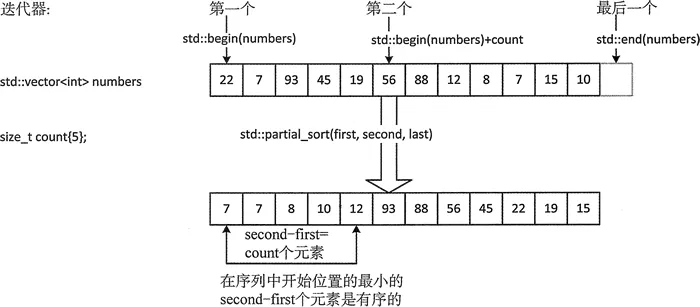# 四、“partial_sort”——STL源码分析

## 4.1、partial_sort 原理

1、首先，对给定集合内区间为[ first , middle ）元素构造大顶堆（make_heap）。

2、其次，遍历剩余区间[ middle , last）中的元素，剩余区间的每个元素均与大顶堆的堆顶元素进行比较，若堆顶元素较小，则交换堆顶元素与遍历得到的元素值( pop_heap)，并重新调整该大顶堆以维持该堆为大顶堆 （adjust_heap）。
3、遍历结束后，[ first,middle ）区间内的元素便是排名在前的k个元素，之后再对该堆做一次堆排序( sort_heap )即可得到最后的前k个有序元素的结果。

## 4.2、partial_sort()算法执行步骤详解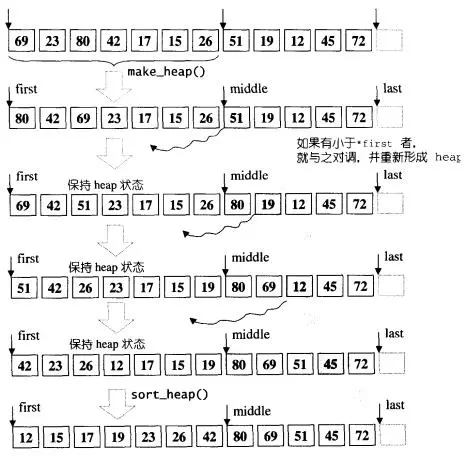## 4.3、Partial_sort方法调用关系图：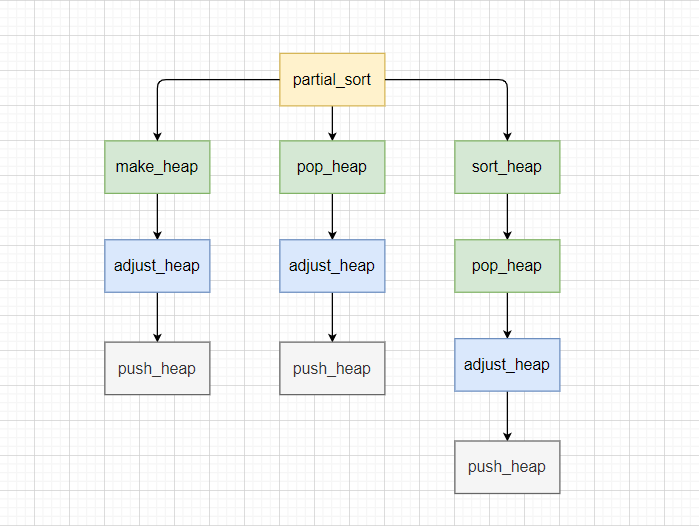## 4.4、C++源码分析

`void __partial_sort(RandomAccessIterator first, RandomAccessIterator middle,  RandomAccessIterator last, T*) {       make_heap(first, middle);  //将区间[first, middle)构造为一个堆结构    for (RandomAccessIterator i = middle; i < last; ++i)      if (*i < *first)     //遍历堆以外的元素，并将更符合要求的元素放入堆中        __pop_heap(first, middle, i, T(*i), distance_type(first)); // first值放i中，i的原值融入heap并调整    sort_heap(first, middle); // 对最终的堆进行排序}`

`make_heap`函数方法（算法）：将一段指定的数据排列为max_heap

`void __make_heap(RandomAccessIterator first, RandomAccessIterator last, T*,  Distance*) {       if (last - first < 2) return; // 如果长度为 0 或 1，不必重新排列      Distance len = last - first;    // 找出第一个需要重新排列的子树头部（即最后一个子树），以parent标记。由于任何叶子节点都不需要处理。    // holeIndex ：标注为需要调整的元素    Distance parent = (len - 2)/2;     while (true) {           // 重排以parent为首的子树，以len为操作范围      __adjust_heap(first, parent, len, T(*(first + parent)));      if (parent == 0) return; // 走完根节点，结束      parent--; // 向前排列前一个节点    }}`

`__adjust_heap`函数方法（算法）：从first开始调整len个元素，holeIndex(洞号)为需要调整的值，其值用value（洞值）存放，最终获得一个max_heap。

`void __adjust_heap(RandomAccessIterator first, Distance holeIndex,  Distance len, T value) {       Distance topIndex = holeIndex;    Distance secondChild = 2 * holeIndex + 2; // holeIndex的右子节点    while (secondChild < len) {         // 比较holeIndex两个子节点的值，用secondChild代表值较大的子节点      if (*(first + secondChild) < *(first + (secondChild - 1)))        secondChild--;         // 令较大子值为洞值，注意：原已在函数形参value中得以保存      *(first + holeIndex) = *(first + secondChild);       // 再让洞号下移到左子节点处，      holeIndex = secondChild;      // 算出新的洞节点的右子节点      secondChild = 2 * (secondChild + 1);    }    // 如果没有右子节点，只有左子节点    if (secondChild == len) {          // 令左子节点为洞值，然后将洞号下移到左子节点      *(first + holeIndex) = *(first + (secondChild - 1));      holeIndex = secondChild - 1;    }    // 将原洞值push到新的洞号中。    // 以下语句的效果类似于：*(first + holeIndex) = value;     __push_heap(first, holeIndex, topIndex, value);}`

`__push_heap`函数方法（算法）：实现将新元素value push到max_heap中 [ topIndex,holeIndex ]的合适位置中，其中max_heap的起始位置为first。

`void __push_heap(RandomAccessIterator first, Distance holeIndex,  Distance topIndex, T value) {       Distance parent = (holeIndex - 1) / 2;  // 找到父节点    // 当尚未达到顶端， 且父节点的值小于新值（不符合max-heap的次序特性）    while (holeIndex > topIndex && *(first + parent) < value) {         *(first + holeIndex) = *(first + parent); // 移动父值到洞号处      holeIndex = parent; // 调整洞号为父节点      parent = (holeIndex - 1) / 2; // 新洞的父节点    }  // 循环到顶端，或者满足max-heap的顺序为止    *(first + holeIndex) = value; // 将新值放入循环完得到的洞号，完成push操作}`

`__pop_heap`函数方法（算法）：互相交换在heap中所指的元素

`inline void __pop_heap(RandomAccessIterator first, RandomAccessIterator last,                       RandomAccessIterator result, T value, Distance*) {     *result = *first; // 将heap顶端元素值放入 result中  // 重新调整heap，洞号为0，欲调整的新值为value  __adjust_heap(first, Distance(0), Distance(last - first), value);}`

`sort_heap`函数方法（算法）：将[ first , middle)中的元素由堆序变为增序排序。每次弹出堆的最大值并放入尾部，然后缩小堆的范围，循环执行弹出操作直到堆只剩下最后一个元素。

`void sort_heap(RandomAccessIterator first, RandomAccessIterator last) {     while (last - first > 1)  // 直到只剩一个元素为止    pop_heap(first, last--); // 每执行一次，范围缩小一格}inline void pop_heap(RandomAccessIterator first, RandomAccessIterator last) {     __pop_heap_aux(first, last, value_type(first));} inline void __pop_heap_aux(RandomAccessIterator first,  RandomAccessIterator last, T*) {       // 将first元素值（即最大值）放入last-1，然后重调[first, last-1)为max-heap    __pop_heap(first, last-1, last-1, T(*(last-1)), distance_type(first));}`

## 4.5、Java模拟实现 partial_sort()

`partial_sort`函数：

`// partial_sort 函数  public boolean partial_sort(T[] t,int nSize) {       int total = t.length;    make_heap(t,nSize); //建立大顶堆    for(int i=nSize;i`

`make_heap`函数：

`// make_heap函数  public boolean make_heap(T[] t,int nSize) {       if(nSize>=2) {    //确保至少有两个元素      for(int i=nSize/2;i>0;) {           --i;        adjust_heap(t,i,nSize);      }    }    return true;  }`

`adjust_heap`函数：

`// adjust_heap 函数  public boolean adjust_heap(T[] t,int pos,int nSize) {       int j=pos;    T v = t[pos];    int k = 2*pos+2;    for(;k`

`sort_heap`函数:

`//sort_heap 函数  public boolean sort_heap(T[] t, int l) {       for(;l>1;--l) {         pop_heap(t,l-1,l-1);    }    return true;  }`

`pop_heap`函数：

`//pop_heap 函数  public boolean pop_heap(T[] t,int m,int i) {       swap(t,0,i); //交换两集合元素    adjust_heap(t,0,m);    return true;  }`

`push_heap`函数：

`//push_heap 函数Java实现  public boolean push_heap(T[] t , int h , int j ,T v) {       for(int i=(h-1)/2;j`

`Comparator`比较器： 定义泛型比较器

`public class Comparator extends AbstractIComparator       {     public boolean equal(Integer x, Integer y) {       if(x == y)      return true;    return false;  }  public boolean less(Integer x, Integer y) {       if(x < y)      return true;    return false;  }}   `

`public class Test {     public static void main(String[] args) {       IComparator        cmp = new Comparator();    Algorithm          obj = new Algorithm           (cmp);    Integer[] t = {   12,9,0,11,29,3,91,20,5,44};    obj.partial_sort(t, 5);    System.out.print("排序后顺序为：");    for(int i=0;i<10;i++) {         System.out.print(t[i]+" ");    }  }}            `# 参考文章

 STL之partial_sort算法源码讲解:https://blog.csdn.net/ggq89/article/details/88817085

 《Java设计模式深入研究》

#### 最新留言

[***.104.42.241]2023年11月13日 19时28分26秒